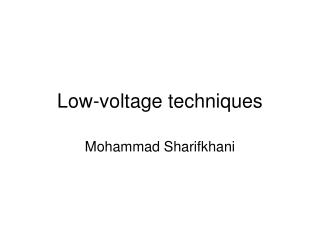# Low-voltage techniques - PowerPoint PPT PresentationDownload PresentationLow-voltage techniques

Low-voltage techniquesDownload Presentation## Low-voltage techniques

- - - - - - - - - - - - - - - - - - - - - - - - - - - E N D - - - - - - - - - - - - - - - - - - - - - - - - - - -
##### Presentation Transcript

1. Low-voltage techniques Mohammad Sharifkhani

2. Reading • Text Book I, Chapter 4 • Text Book II, Section 11.7

3. Power, Energy, Speed • Speed • Energy  Battery lifetime • Instantaneous power  Package, cooling If leakage is ignored, P x Tpd is equal to E; independent of Vth and Speed: work at the slowest speed; lowest VDD to minimize E (and P).

4. Efficient design approaches

5. Power, Energy, Speed • Both Energy and Speed are important: • Energy x Delay is the right index (?) • To minimize power • Lower VDD (quadratic dependence, +both leakage and dynamic power) • Reduce C • Lower pt • Lower VDD  Delay  4 possibilities • Dual Vth (low Vth only for critical path) • Multiple VDD (low VDD for non critical path) • Parallel, pipeline arch. • Lower Vth to recover the speed

6. VDD scaling

7. VDD scaling

8. VDD scaling

9. VDD scaling vs. delay

10. Processing options

11. Parallel data path

12. Pipeline data path

13. Comparison

14. Multiple supply issues Still on!  DC current

15. Block level voltage scaling

16. Block level multiple supply voltage

17. Multiple VDDs

18. Optimum V2/V1 is around 0.7V Hamada, CICC’01

19. Multiple supply voltages • Two supply voltages per block are optimal • Optimal ratio between the supply voltages is 0.7 • Level conversion is performed on the voltage boundary, using a level-converting flip-flop (LCFF) • An option is to use an asynchronous (combinatorial) level converter • More sensitive to coupling and supply noise

20. Level converting FF

21. Shimazaki, ISSCC’03

22. VDDH drives

23. Inverse discrete cosine

24. Delay sensitivity

25. VDD temporal variation • Design for Dynamically Varying VDD • • Ring oscillator. • • static logic • • Dynamic logic (& tri-state busses). • • Sense amp (& memory cell). • Max. allowed |dVDD/dt| → Min. CDD = 100nF (0.6μm) • Circuits continue to properly operate as VDD changes VDD t

26. Static CMOS